It is a strategy for fixed-income investors looking to reduce price sensitivity in their portfolio as a whole due to interest rate risk

Developing a bond ladder is a strategy for fixed-income investors looking to reduce price sensitivity in their portfolios due to interest rate risk. Creating a ladder is also a strategy to structure cash flow more consistently.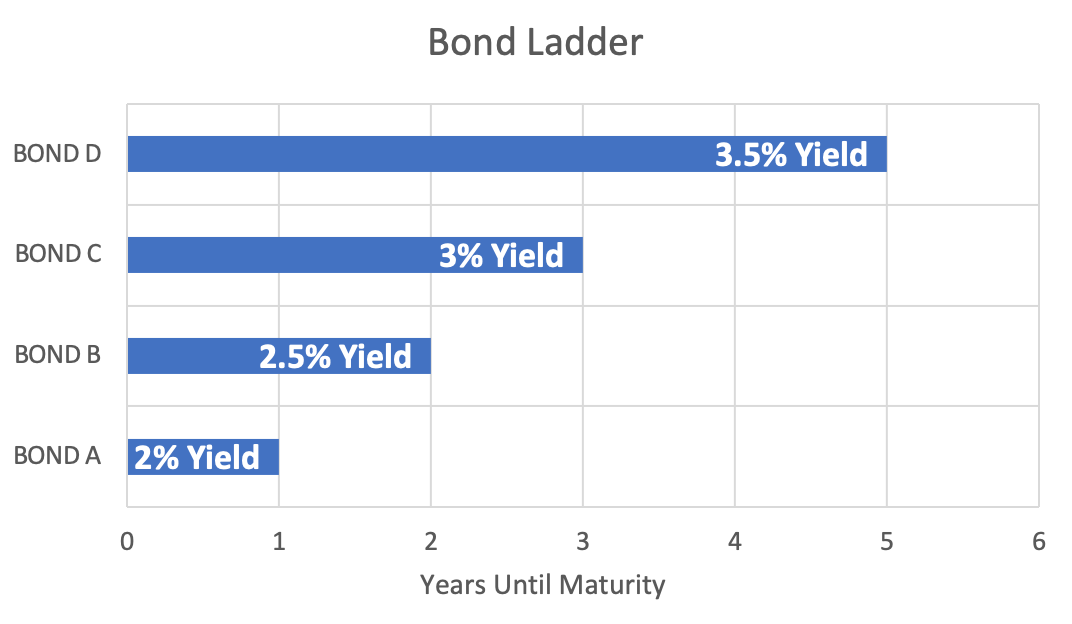There are a few key ideas to understand why this strategy is effective:

1. By investing in bonds that mature at different dates rather than multiple bonds that develop during the same year, changes in interest rates will not affect the entire portfolio equally. This is because price sensitivity depends on the maturity of a bond.
2. By staggering bond maturity dates, principal repayments are more frequent than if the bonds were to mature simultaneously. This gives investors more flexibility and locks up more significant portions of capital for shorter periods.

These core concepts are based on the specifics of a bond's payment structure as it reaches maturity and how investors calculate bond valuation

## Bond Structure and Valuation

Bonds are an example of a fixed income asset with specified payments in the contract. For example, a bond contract includes the par-value (also known as the principal), coupon rate, maturity, and specifications on repayment frequency.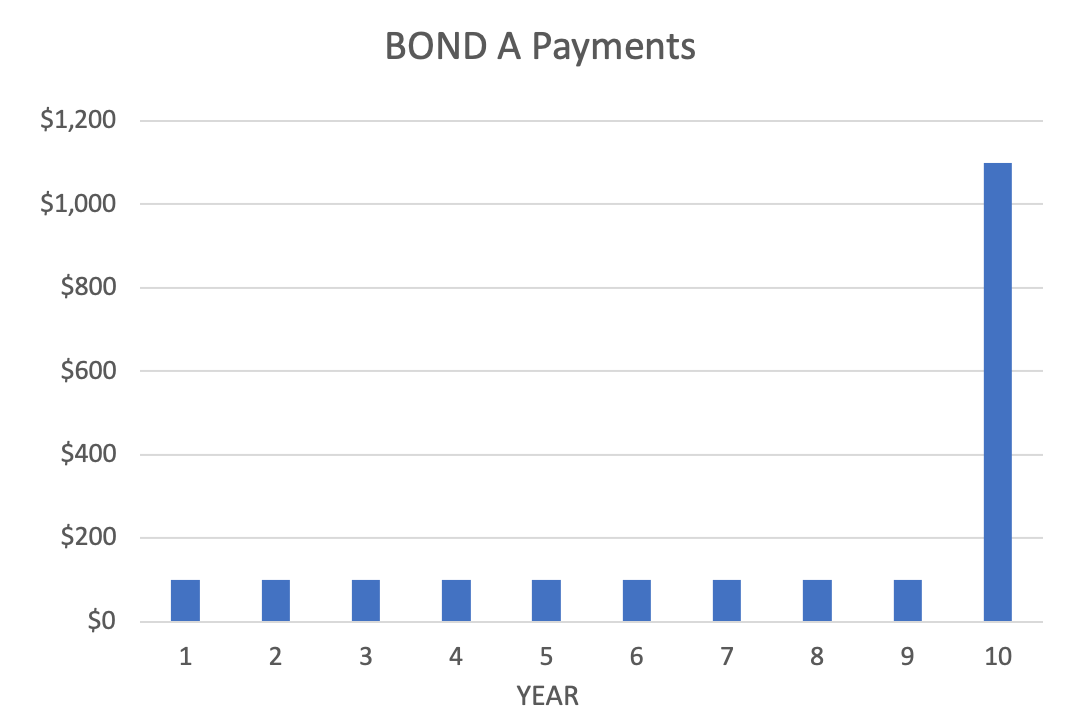The par-value is the amount of money an individual loans the bond issuer. Examples include governments and corporations issuing bonds to finance expenditures with debt. The principal is repaid once the bond matures, along with the final coupon payment.

The coupon rate determines the amount of money each coupon or a fixed payment is worth. For example, a 5% coupon on a bond with a principal of \$1000 would be \$50.

Coupon Payment = Coupon Rate x Principal

The maturity is the time until the principal is repaid to the investor. For example, a maturity of 7 years would mean that the investor can expect coupon payments for the next seven years, along with the par-value.

Repayment frequency is how often the investor will receive coupon payments. The coupon payment is typically annual but can also be semi-annual or quarterly. In this case, the coupon payment would be divided by the number of periods per year.

Coupon Payment = (Coupon Rate / Periods per Year) x Principal

As is the case with valuing future returns for most assets, we must consider the time value of money to determine the price of a bond.

If you ask anyone, the decision between receiving \$100 now vs. \$100 in ten years is quite simple. Although the money now is sure, there is no guarantee of receiving money ten years from now.In addition to the uncertainty of the future, inflation and interest rates reduce the present value of money in the future. For example, we assume a 2% annual inflation rate, that \$100 has 2% less purchasing power each year.

This means for each year into the future, your money can buy fewer products, even if you have the same nominal dollar amount!

To get around this problem, we discount the future payments to determine their present value with the following equation:

Present Value = Future Value/(1 + r)n

We know that a bond offers a series of fixed payments over time. The bond's present value is the sum of the current value of all these payments. This is typically synonymous with the price of the bond.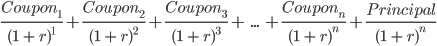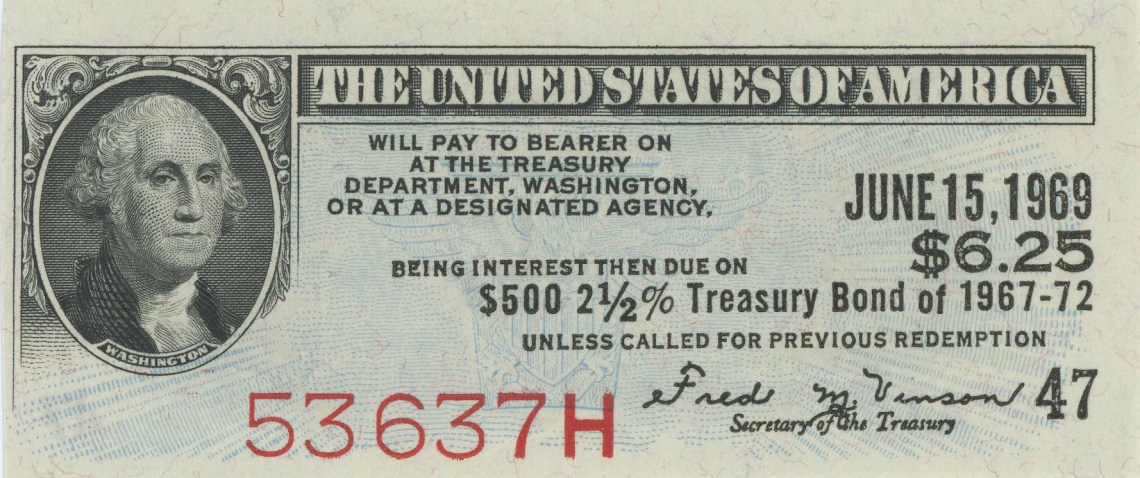If the bond price were higher than the value of those payments, people would not buy, lowering the price. But conversely, if the price were lower than the value of those payments, the cost would rise with the increase in demand.

This leaves one important question. What value should be used for the discount rate, allowing us to solve for the present value? Investors' required rate of return determines the discount rate.

This is because the discount rate is equivalent to the yield to maturity or annual return on the investment if held until the bond matures.

How do investors formulate their required rate of return? First, investors think about what rate of return they can get without any risk and then add premiums for any added risks the investment has.

Required Rate of Return = Risk-free Rate + Risk Premiums

If there were no premiums for added risk, investors would opt not to invest. Instead, they would invest in the less risky alternative, offering the same return without added risk.

A few examples of risk premiums are essential for determining the required rate of return in a bond. These include:For bonds more likely to default, coupon payments and the principal repayment are deemed less specific. As a result, a premium for this added risk is called the default risk premium. The difference in bond yield concerning default risk is called the credit spread.

The maturity risk premium is associated with the time until maturity. This is because a more extended period until maturity means more time for interest rates to change, impacting the risk-free rate. Why does this matter?

Changes in the risk-free rate affect the entire yield curve and can make a bond lose value, as newer bonds offer a higher yield for less risk. To capture interest-rate sensitivity, this premium is also included in the price of a bind.

Liquidity risk is a premium added to bonds that do not have an ample supply or demand. As a result, exiting the position is difficult, especially when default seems likely. For this reason, an extra premium is added.

## Why does it work?

Understanding bond pricing and its relation to discount rates give us the core tools to appreciate why a bond ladder is an effective strategy.

The main takeaway is that creating a ladder removes the impact of maturity length on price sensitivity from changing interest rates.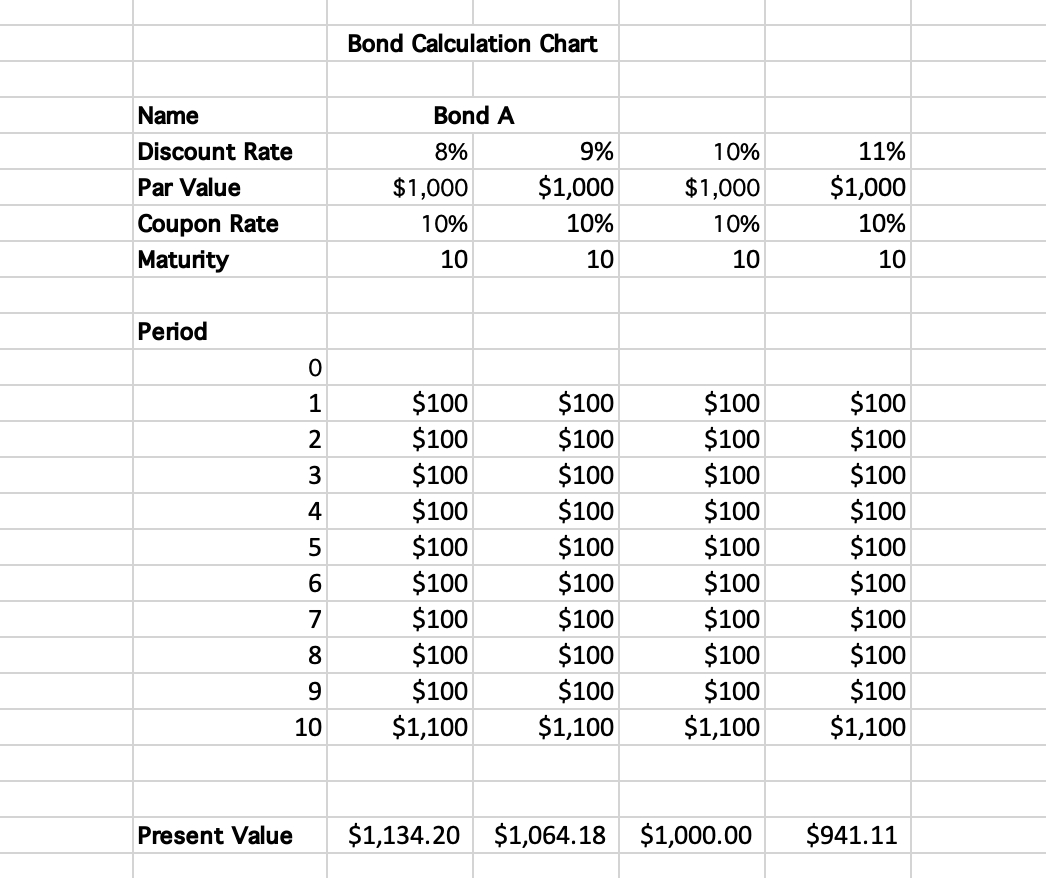Looking at our formula for determining a bond's price, we see that the discount rate is in the denominator of all the terms we add. Therefore, an increase in the discount rate would lower the price, while a rise in the discount rate would reduce costs.

To make the comparison simple, let's compare two portfolios of bonds. The first portfolio has a group of bonds, all expiring in 10 years, while the second portfolio has bonds expiring in 1 year, five years, and ten years.

How do the prices of the two bond portfolios compare with changes in interest rates? Let's say the central bank increases the target for the federal funds rate, raising it by 100 basis points. At this point, the entire yield curve would be shifted by 1% as the risk-free rate increases.

Because the risk-free rate increases, the investor's required rate of return also increases by 1%. This means that the present value of future cash flows is now lower than before the rate hike, as we are discounting them more steeply.

The effect of this discount is much more significant for the first portfolio, as all of the bonds are maturing further in the future. This is because of the compounding effect of discounting as given by the present value formula.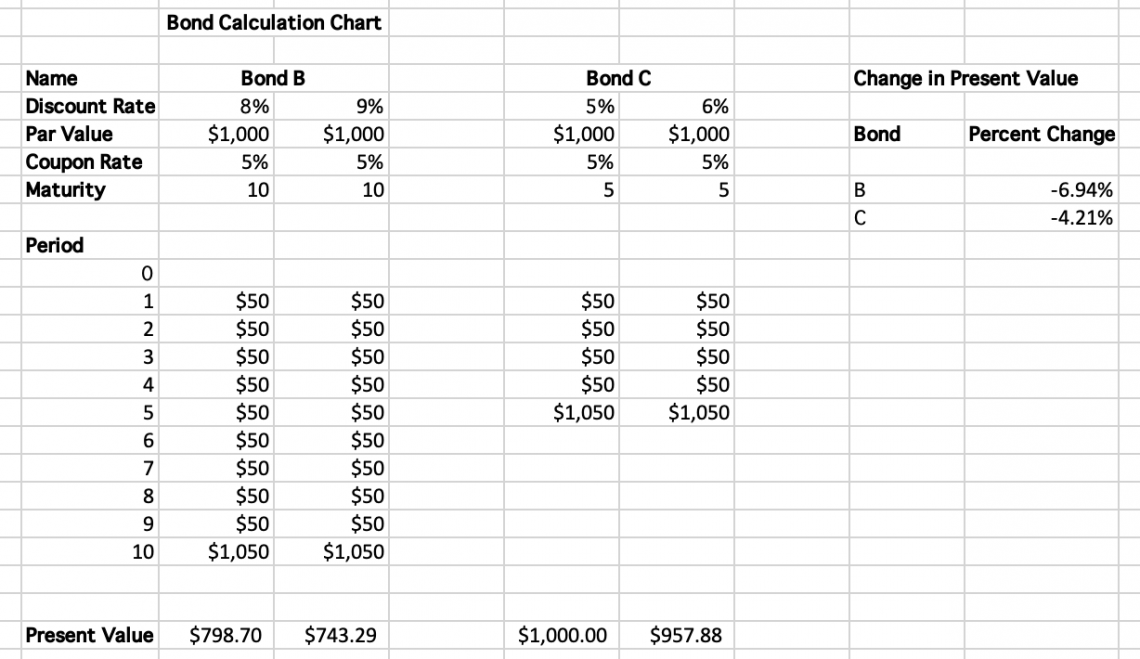Compare the discount on the principal repayment for both of these bonds: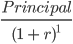VS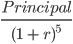Assuming the principal repayment and the discount rates are the same, we can see the discounting effect is more influential on the present value of the bond expiring in 10 years. This is what is known as interest rate sensitivity.

Because the first portfolio comprises bonds expiring in 10 years, the price will shift dramatically for all bonds. However, in the second portfolio, the impact on the cost of changing rates will be less profound, as some bonds are closer to maturity than those in the 10-year maturity grouping.

The thinking behind creating a bond ladder is that it smoothes out the fluctuations in interest rates in the portfolio.

For bonds close to maturity, we can purchase new long-term bonds offering higher yields with principal repayments due to increased interest rates. This helps to offset losses in the value of existing long-term bonds, which just decreased in value from rising interest rates.

The thinking is the same for when interest rates decline. New bond purchases will have lower yields. However, longer-term bonds in the portfolio will have increased in value.

Essentially, we will have more frequent fixed payments by creating a ladder. This allows us to reinvest at higher rates when our portfolio declines and see capital gains when yields fall.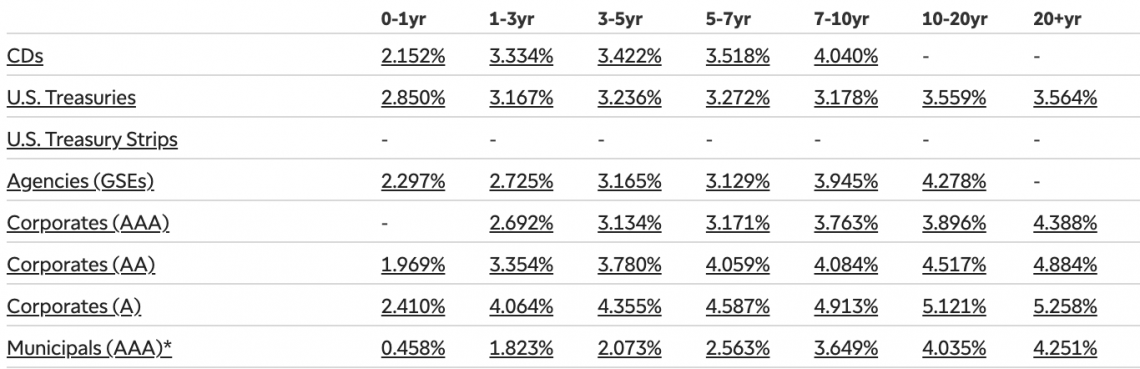The portfolio's overall yield will be the average of the yields locked in at different investment times, offering a more consistent return to investors.

Another positive of a bond ladder's more consistent cash flow is the ability to take advantage of other opportunities.

As in portfolio one, if an investor has only a portfolio of long-term bonds, they will have their money locked up until maturity if they want to keep the rate of return from the time of their investment without having to worry about the possibility of capital losses.

When creating a ladder, there are a few different components to consider. These will impact income generation and the risk associated with the ladder.

These include:

• Rungs
• Spacing
• Material

The ladder rungs are the different coupon payment dates and maturity dates. The number of rungs is determined by investor preference and their chosen investment amount.

For an investor looking to invest \$10,000, they can create ten rungs of equal portions of \$1000. It is also possible to have rungs of different weights, although this could increase risk.

Considerations some investors have when creating rungs are coupon and maturity dates. By staggering the dates of these rungs, coupon payments become more consistent.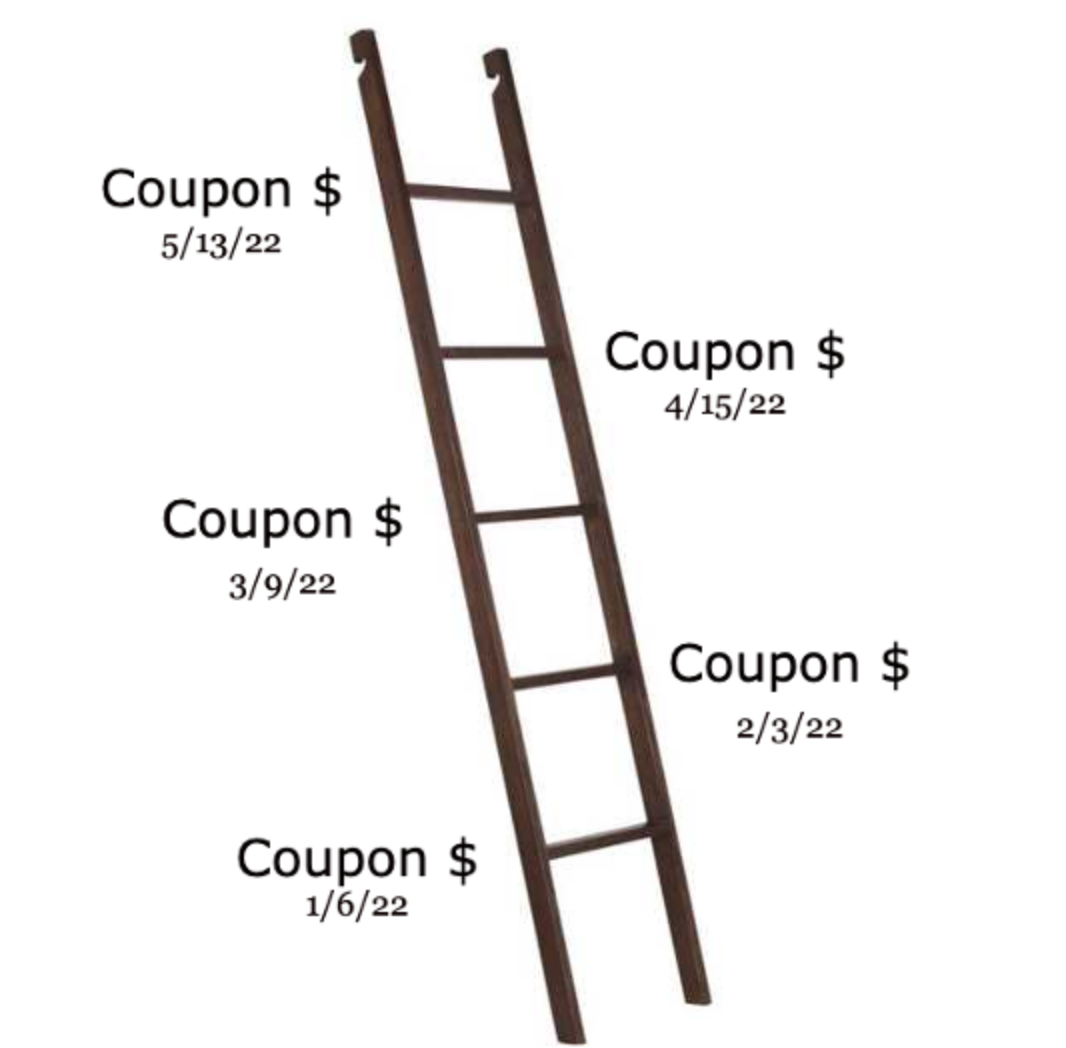For example, with just six semi-annual coupon payment bonds, it is possible to have a monthly income from coupon payments. Spacing refers to how far apart the bond maturities are. Equal spacing is considered to be the less risky option, but once again, it is up to investor preference.

With bonds spaced further apart, investors can take advantage of the higher yields of longer-term bonds. The downside to this is less consistent principal repayment.

The material of the bonds is precise about what type of bonds are being invested in. Because creating a bond ladder is a risk management tool, it is common for investors to choose investment-grade bonds compared to risky bonds with a higher likelihood of default.### Everything You Need To Master Excel Modeling

To Help You Thrive in the Most Prestigious Jobs on Wall Street.

Researched and authored by Jacob Rounds | LinkedIn

Reviewed and edited by Tanay Gehi | Linkedin